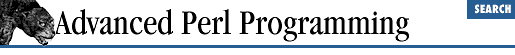home | O'Reilly's CD bookshelfs | FreeBSD | Linux | Cisco | Cisco Exam

### 2.5 Pretty-Printing

In building complicated data structures, it is always nice to have a pretty-printer handy for debugging. There are at least two options for pretty-printing data structures. The first is the Perl debugger itself. It uses a function called ``` dumpValue``` in a file called dumpvar.pl , which can be found in the standard library directory. We can help ourselves to it, with the caveat that it is an unadvertised function and could change someday. To pretty-print this structure, for example:

`  @sample = (11.233,{3 => 4, "hello" => [6,7]});`

we write the following:

```require 'dumpvar.pl';
dumpValue(\@sample); # always pass by reference```

This prints something like this:

```0  11.233
1  HASH(0xb75dc0)
3 => 4
'hello' => ARRAY(0xc70858)
0  6
1  7```

We will cover the require statement in Chapter 6, Modules . Meanwhile, just think of it as a fancy #include (which doesn't load the file if it is already loaded).

The Data::Dumper module available from CPAN is another viable alternative for pretty-printing. Chapter 10, Persistence , covers this module in some detail, so we will not say any more about it here. Both modules detect circular references and handle subroutine and glob references.

It is fun and instructive to write a pretty-printer ourselves. Example 2.5 illustrates a simple effort, which accounts for circular references but doesn't follow typeglobs or subroutine references. This example is used as follows:

```pretty_print(@sample); # Doesn't ```

need
```
a reference```

This prints

```11.233
{ # HASH(0xb78b00)
:  3 => 4
:  hello =>
:  :  [ # ARRAY(0xc70858)
:  :  :  6
:  :  :  7
:  :  ]
}```

The following code contains specialized procedures ( print_array , print_hash , or print_scalar ) that know how to print specific data types. print_ref , charged with the task of pretty-printing a reference, simply dispatches control to one of the above procedures depending upon the type of argument given to it. In turn, these procedures may call ``` print_ref``` recursively if the data types that they handle contain one or more references.

Whenever a reference is encountered, it is also checked with a hash %already_seen to determine whether the reference has been printed before. This prevents the routine from going into an infinite loop in the presence of circular references. All functions manipulate the global variable \$level and call print_indented , which appropriately indents and prints the string given to it.

#### Example 2.5: Pretty-Printing

```\$level = -1; # Level of indentation

sub pretty_print {
my \$var;
foreach \$var (@_) {
if (ref (\$var)) {
print_ref(\$var);
} else {
print_scalar(\$var);
}
}
}

sub print_scalar {
++\$level;
my \$var = shift;
print_indented (\$var);
--\$level;
}

sub print_ref {
my \$r = shift;
print_indented ("\$r (Seen earlier)");
return;
} else {
}
my \$ref_type = ref(\$r);
if (\$ref_type eq "ARRAY") {
print_array(\$r);
} elsif (\$ref_type eq "SCALAR") {
print "Ref -> \$r";
print_scalar(\$\$r);
} elsif (\$ref_type eq "HASH") {
print_hash(\$r);
} elsif (\$ref_type eq "REF") {
++\$level;
print_indented("Ref -> (\$r)");
print_ref(\$\$r);
--\$level;
} else {
print_indented ("\$ref_type (not supported)");
}
}

sub print_array {
my (\$r_array) = @_;
++\$level;
print_indented ("[ # \$r_array");
foreach \$var (@\$r_array) {
if (ref (\$var)) {
print_ref(\$var);
} else {
print_scalar(\$var);
}
}
print_indented ("]");
--\$level;
}

sub print_hash {
my(\$r_hash) = @_;
my(\$key, \$val);
++\$level;
print_indented ("{ # \$r_hash");
while ((\$key, \$val) = each %\$r_hash) {
\$val = (\$val ? \$val : '""');
++\$level;
if (ref (\$val)) {
print_indented ("\$key => ");
print_ref(\$val);
} else {
print_indented ("\$key => \$val");
}
--\$level;
}
print_indented ("}");
--\$level;
}

sub print_indented {
\$spaces = ":  " x \$level;
print "\${spaces}\$_\n";
}```

print_ref simply prints its argument (a reference) and returns if it has already seen this reference. If you were to read the output produced by this code, you would find it hard to imagine which reference points to which structure. As an exercise, you might try producing a better pretty-printer, which identifies appropriate structures by easily identifiable numeric labels like this:

```:  hello =>
:  :  [          # 10
:  :  :  6
:  :  :  7
:  :  ]
:  foobar => array-ref # 10
}```

The number 10 is an automatically generated label, which is more easily identifiable than something like ARRAY(0xc70858) .2.4 Pass the Envelope2.6 Resources